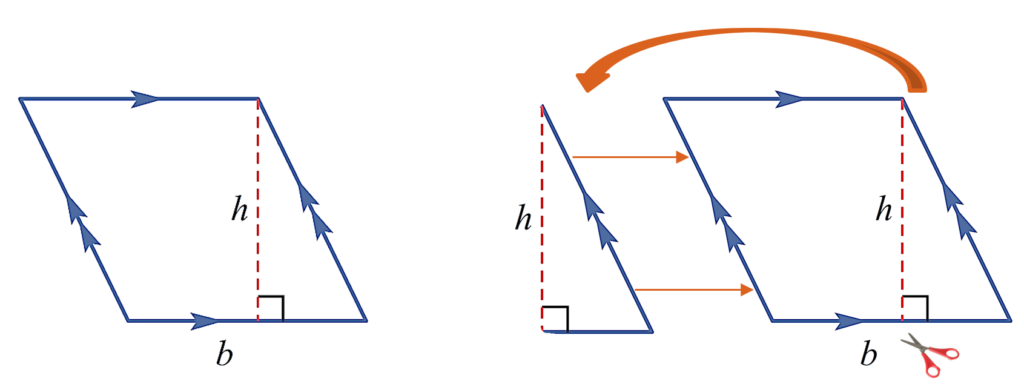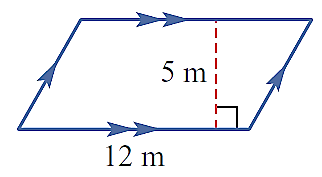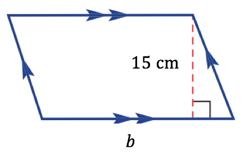PRIMARY

2 - 6

SECONDARY

7 - 10

SENIOR

11 - 12

Home

# Parallelogram

Theory & Practice Questions.

## Lesson

To develop a formula to find the area of a parallelogram, cut a triangle from one end of the parallelogram and shift it to the opposite side of the parallelogram to make a rectangle.

Then use the following formula:

 A = b x h## Example

Find the area of the parallelogram.## Explanation

Area of a parallelogram = base of the parallelogram  the perpendicular height

A = bh

Substitute given values into the formula.

A= 5x12

A = 60m²

## Question

Find the base length of the parallelogram if the area is equal to 67.5cm²## Explanation

Recall the formula for the area of a parallelogram.

A = b x h

Using this.

67.5 = x 15

4.5 = b

Therefore, the base of the parallelogram is equal to 4.5cm.